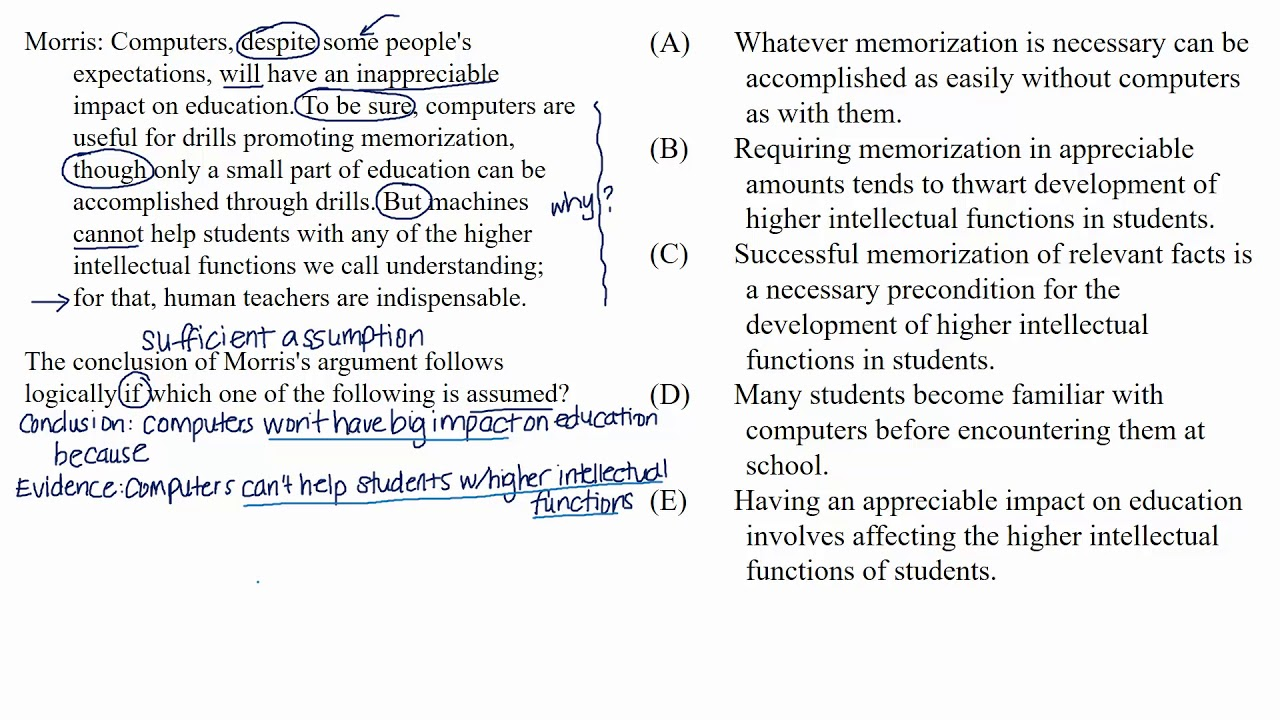On the GMAT, you must be able to identify any assumptions, or gaps, between the evidence and the conclusion. There are two types of Assumptions: Sufficient Assumptions and Necessary Assumptions. These questions typically ask you to identify the flaw and fix it. So, these are often like the Strengthening/Weakening questions we just discussed.

### CHALLENGE: Identify the Necessary/Sufficient Assumptions

Necessary Assumption questions have stems phrased in the following ways:

• The argument requires/depends on/relies on the assumption that…
• Which of the following is an assumption on which the argument relies/depends?
• Which one of the following is an assumption the argument requires?
• The argument requires/depends on/relies on assuming which one of the following?

Sufficient Assumption questions are phrased like this:

• The conclusion follows logically if which one of the following is assumed?
• The conclusion of the argument can be properly drawn if which one of the following is assumed?

Notice that Necessary Assumption questions always contain a word that means “necessary” or “required”, while Sufficient Assumption questions ask for a guarantee that the conclusion will be reached.#### Sufficient/Necessary

Best viewed in landscape mode

3 questions with video explanations

100 seconds per question